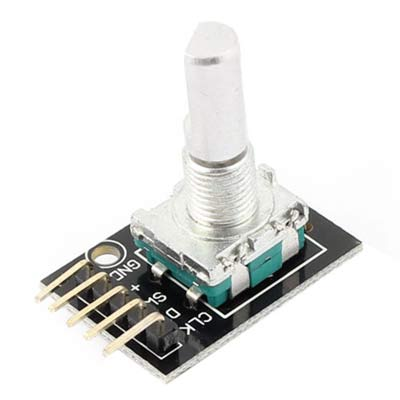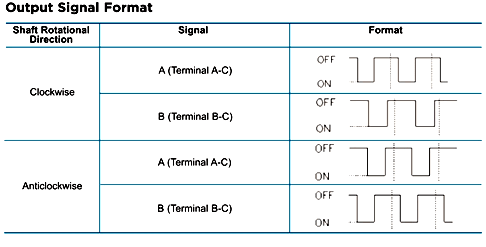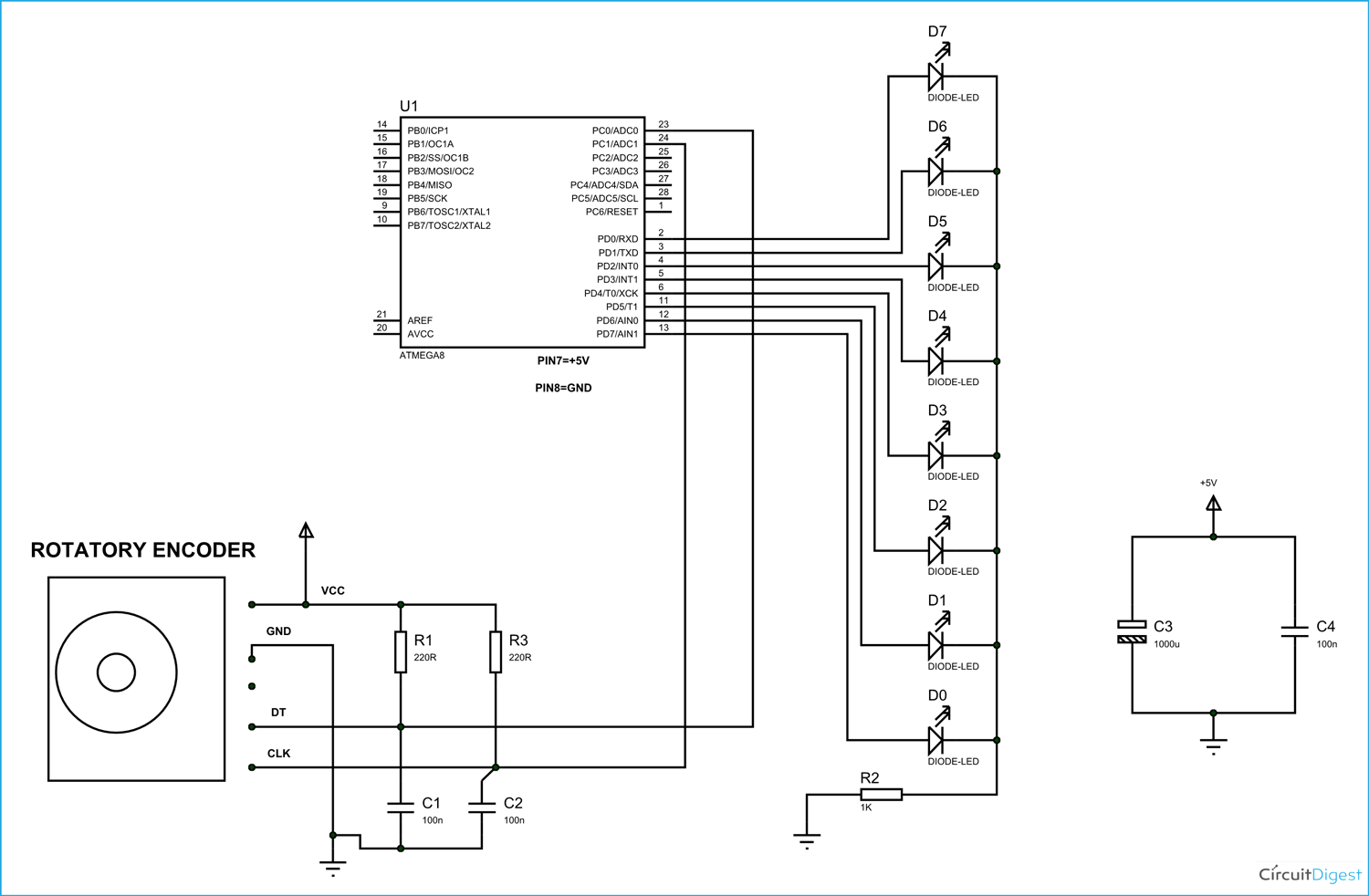In this tutorial we are going to interface a rotary encoder with ATmega8 microcontroller.  ATmega8 is atmel's microcontroller series designed for embedded applications. Rotary encoder is used to know the position of movement and angular movement of a motor or axis. It’s a three terminal device usually, with power and ground pin counts to five. A rotary encoder module is shown below.The pins of a rotary encoder are:

• Ground.
• Positive connected to +5V
• Output signal A pin
• Output signal B pin
• Clock pin or common pin.

The ENCODER provides pulses representing the change in the shaft position for the systems to understand. Consider a ROTATORY ENCODER is powered up and the shaft is moved.As shown in above table, consider the shaft is moved clock wise. With this there will be Falling Edge at the A terminal then at B terminal.

Consider the shaft is moved Anti clock wise. With this there will be Falling Edge at the B terminal then at A terminal.

This edge will occur once for 360/20 = 18 degrees (This is for a Encoder with 20 position, this changes from type to type, higher the count greater the accuracy).

With both above conditions, we can get direction and degree of rotation. Thus we get required parameters for getting the position of a shaft.

### Components

Hardware: ATMEGA8, connecting pins, AVR-ISP PROGRAMMER, 220Ω resistor, LED (eight pieces), 1KΩ resistor, 220Ω resistor (2pieces), 100nF capacitor (2 pieces ), bread board.

Software: Atmel studio 6.1, progisp or flash magic.

### Circuit Diagram and Working ExplanationThe capacitors here are for neutralizing the contact bouncing effect in ENCODER. Without those capacitors there will be some serious problems in position reading.

When the shaft is moved there will be time difference between two output pins output. The ATMEGA8 will recognize this time difference for clockwise of Anti clock wise rotation.

If the rotation is clockwise the binary count is incremented by one, and this count is shown in LED port as shown in figure.

If the rotation is Anti clock wise the binary count is decremented by one and the binary count is shown at LED byte.

Remember here we are not setting any fuse bits of atmega8, So the controller will be working on 1Mhz default internal crystal.

Working of interfacing rotary encoder with ATmega8 microcontroller is explained in below code.

Code

#include <avr/io.h>

// header to enable data flow control over pins

#define F_CPU 1000000UL

//telling controller crystal frequency attached

#include <util/delay.h>

//header to enable delay function in program

int main(void)

{

DDRD = 0xFF;// portD as output

PORTD =0;

DDRC= 0;//portC as input

int count =0;

int i=0;

while(1)

{

if (i==0)

{

if ((bit_is_clear(PINC,0))||(bit_is_clear(PINC,1)))

//If any Encoder pins show falling edge execute lop

{

i= 1;

_delay_ms(10);

if (bit_is_clear(PINC,0) )//if PINB is second to go LOW

{

if (count<255)

{

count++;

// increment binary count if count is less than 255

}

}

if (bit_is_clear(PINC,1))// if PINA is second to go LOW

{

if (count>0)

{

count--;

// if binary count is greater than 0 decrease count by 1

}

}

}

}

PORTD = count;

if ((bit_is_set(PINC,0))&&(bit_is_set(PINC,1) ))// wait till shaft position reset

{

i = 0;

}

}

}

Video

Subscribe below to receive most popular news, articles and DIY projects from Circuit Digest

### Related Content

•Saeed Mahdavi Asl
Jun 11, 2016

it is good for beginners and good to start working with microcontrollers
be happy
Saeed Mahdavi Asl

•Harshal
Jul 10, 2016

What should if I want digital output on the 16×2 LCD display